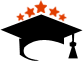Updated On - May 30th 2023, Updated By - ishika

# How to convert CGPA to Percentage?

CGPA to Percentage: To convert CGPA to Percentage is as simple as finding percentage of marks from the grading system,Some of them follow a grading system whereas some state boards follow the Percentage pattern.in such cases, students want to know How to Calculate CGPA into Percentages? Or How to convert CGPA to Percentage? Because universities ask to fill the scores in percentage form.  If they want to fill out any admission form of any university, their students need to convert their CGPA Into a percentage. 9.5 Grading scale is used most commonly in Schools if India.

It is possible how to convert CGPA to a percentage by determining the weighted percentage of grades. In schools and colleges, students are graded on a scale from A+ to F, and they want to know how they convert CGPA to percentage, which is expressed on this scale. The cumulative grade point average referred to as a CGPA is converted to a percentage based on the average grade received across all subjects according to the study plan.

Students may find several online platforms to help them convert their CGPA to a percentage. Better yet, students may apply to several institutions using the quantitative value of their percentage marks. India uses a percentage-based grading system, which differs from country to country according to different institutional structures and processes.

In CBSE more than 50% of students are awarded an 8.2 CGPA. It means they get B2 grade. Students who come in the category of below average, mostly get 4 CGPA. It indicates that they have qualified and been promoted to the next class. But students who get less than a D grade or 4 CGPA. Such students will have to revise their classes.

8.2 cgpa in percentage is written as 77.90% in CBSE. Do you know that? So Many Free tools are available on the internet to check CGPA to Percentage, also called CGPA to Percentage calculator. But all tools are not showing the same output; that's a big problem, so always trusted top brand CGPA Calculator tools to check the value in Percentage for your grading system.

CGPA to Percentage is also an important thing for a Group of Students who want to take Admission in Diploma Courses after 10th and Diploma Courses after 12th

Science Courses after 12th Multiple methods we shared below in this article to covert CGPA in Percentage. One can read it and get the important information.

## What is CGPA?

The grading system is new marks measuring scale in the Indian Education culture. Earlier, Students used to get marks in each subject. But now, In schools teachers assign Grades to each student. It is a cumulative mark. It includes not only the answers written on the answer sheet. A student taking active participation in other activities and in other co-curricular activities is also been noted by the teachers.

Calculate and add the grade points for each subject in a semester, then divide by the total number of subjects to get the CGPA. Now we know what CGPA is, and then we'd know how to convert CGPA to Percentage

Many students search for a 4.0 GPA Percentage. They can check the CGPA in the percentage table that is given below. Such students who get an SGPA of 4, have scored 38% on their scorecards.

Commonly students achieve 8.2 to 9 grades on their report cards. If they won't learn to convert 8.2 cgpa in percentage then the Conversion chart is given below.

### CGPA to percentage conversion certificate

The term "CGPA to percentage conversion certificate" refers to a document that takes an individual student's cumulative grade point average (CGPA) and turns it into a percentage. Universities and other types of institutions often require applicants to provide this certificate along with their admissions applications.

The conversion from CGPA to percentage is not an exact science. The cumulative grade point average (CGPA) cannot be converted to a percentage using a single formula that is applicable to all educational institutions. The grading system that an institution opts to use will likely result in a modification to the conversion formula.

### how to convert cgpa into marks

Nowadays Universities and colleges calculate the performance of students using grade points. They have started using the CGPA method to calculate the student's marks. There is an example of how to convert CGPA into marks,if you need to understand how to convert CGPA to percentage? you have to multiply your CGPA9.5. for example if you got an 8 CGPA your total percentage of marks would be 89.5= 76%. Furthermore, if you want to calculate total marks you can multiply your percentage and divide by 100 by the total marks (total subjects). Here is an example if you have a total of 5 subjects, all of the subjects have individual 100 marks, your marks in 12th Grade would be (76/100)*500= 380 marks.

## How to Convert CGPA to Percentage?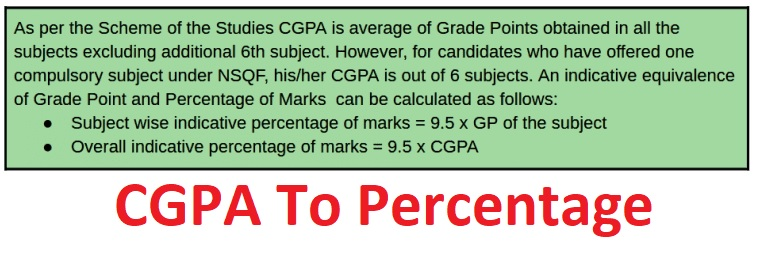In the Grading system, students did not get the exact marks in any subjects. Students who have a difference of 4-8 marks are categorized in the same slot and get grade Points. These grades are used to minimize competition among students.

In 2011, CBSE stopped using the CGPA grading system to give marks. You can compute your percentage from your grade using an online CGPA to percentage converter. The process of applying standardised measurements of various levels of accomplishment in a course is known as grading in education.

When students pass their matriculation(10th) exams, they can pursue many diploma certificate courses. Then, students require to know how to convert CGPA to a percentage because many educational institutes release merit lists for admission. Grade points are assigned for every grade. Grade A1 represents 10 Grade points’. Nine grade points are represented by grade ‘A2’.For getting a percentage from CGPA Just, multiply the grade point to 9.5, and the answer is the Percentage. For example, A student gets a total CGPA grade of 10 on his report card. The Percentage will be 10*9.5= 95%.

Converting CGPA to Percentage is very easy. If you want to get the overall estimated Percentage, then all you need to do is multiply your CGPA by 9.5.

Step 1: First of all, note all of your grand points for each subject.

Step 2:Divide the total grade point by the number of subjects. This refers to CGPA. Let you have obtained points 7, 7.5, 8, 8.5, and 9 in five subjects. Initially get their average

Step 3: Now, multiply the CGPA by 9.5, Average CGPA = (7+7.5+8+8.5+9)/5

Step 4: This will result in a percentage. Hence the Average CGPA is = 8

## Difference between CGPA and percentage

The primary difference between CGPA and percentage is that, while both assess performance overall, percentage(%) also measures performance in percentage form the whole semester or unit, whereas CGPA measures performance over the whole course (covering all semesters and units). The following examples will help you understand how to convert CGPA to Percentage?

• Percentage(%) may cover a total performance in decimal form, such as a semester or term, but CGPA must cover a longer time period, such as the entirety of the degree or course (inclusive of all semesters).
• For a superior overall grade point average (CGPA) in your degree course, you should maintain a strong percentage in the whole semester.
• Students' percentage(%) is typically the performance of candidates to get  consistency in academic achievement over the course of any given course. In many cases, they downplay CGPA.
• While universities in the UK and many other countries use the 4-point CGPA system, still ask about the cgpa to percentage institutions in India utilize the 10-point CGPA system.

## Online CGPA Calculator

CGPA to percentage converter: The majority of students struggle to calculate their percentage since they are unfamiliar with the concept of CGPA. Students can use the online CGPA Calculator to get their marks in percentage from CGPA. Knowing the proportion will also assist applicants get into college and advance in their career options.

The cumulative grade point average (CGPA) may be requested from candidates for entrance to higher education or job applications, however for some criteria, students need it changed into a percentage. The online CGPA conversion process may differ depending on the institution or university.

According to the CGPA to percentage converter grading system to calculate in percentage form, many universities use a 4.0 grading scale to calculate its maximum marks whereas the 10.0 grading scale is most commonly used to calculate. The direct approach is a straightforward translation from CGPA/SGPA to %. In the weighted method, specific topics referred to as major subjects will receive increased weightage, which will subsequently be turned into percentages(%). The universities that adhere to their own criteria determine the consistency in calculating CGPA/SGPA into percentages(%).

## CGPA to Percentage CBSE

The board examination results have been disclosed by the Central Board of Secondary Education. The statistics are presented as a cumulative grade point average (CGPA): In accordance with the exam's hierarchy, it is the aggregate of the Grade Points earned in all subjects, excluding the sixth supplementary subject.

First Calculating CGPA

Even though it is listed on the report card, it is simple to figure out your CGPA by yourself. Here is a step-by-step guide to follow.

Subjects 1 through 5 are: 9, 8, 6, 5, and 7, respectively.

Step 2: Divide it by 5.

35/5 = 7.0

It is now crucial to comprehend your outcome in percentages in order to assess your performance.

Secondly Calculating CGPA to Percentage(%)

Multiply your aggregate CGPA by 9.5 to get your CGPA as a Percentage(%).

For instance,

As 7.0 (CGPA) is multiplied by 9.5 in this instance, 66.5% results..

 CGPA Conversion Table 4.0 Scale Percent(%) 4.0            (A) 60-100(%) 3.0-3.9     (B) 50-59(%) 2.0-2.9     (C) 35-49(%) 1.0-1.9     (D) — 0.0-0.9     (F) 0-34(%)

In India, Various Colleges and Universities assess candidates using their grades or percentage. And Some institutions have their own grading schemes, like NITs and IITs. The academic grading system used in India is based on a percentage system (out of 100) or a 4.0 GPA system, unlike the international grading system (GPA/CGPA) used by schools and universities abroad.

## Conversion From CGPA to Percentage Table (9.5 Grading scale)

 CGPA Percentage 3 CGPA in percentage 28.50% 3.1 CGPA in percentage 29.45% 3.2 CGPA in percentage 30.40% 3.3 CGPA in percentage 31.35% 3.4CGPA in percentage 32.30% 3.5 CGPA in percentage 33.25% 3.6 CGPA in percentage 34.20% 3.7 CGPA in percentage 35.15% 3.8 CGPA in percentage 36.10% 3.9 CGPA in percentage 37.05% 4 CGPA in percentage 38% 4.1 CGPA in percentage 38.95% 4.2 CGPA in percentage 39.90% 4.3 CGPA in percentage 40.85% 4.4 CGPA in percentage 41.80% 4.5 CGPA in percentage 42.75% 4.6 CGPA in percentage 43.70% 4.7 CGPA in percentage 44.65% 4.8 CGPA in percentage 45.60% 4.9 CGPA in percentage 46.55% 5 CGPA in percentage 47.50% 5.1 CGPA in percentage 48.45% 5.2 CGPA in percentage 49.40% 5.3 CGPA in percentage 50.35% 5.4 CGPA in percentage 51.30% 5.5 CGPA in percentage 52.25% 5.6 CGPA in percentage 53.20% 5.7CGPA in percentage 54.15% 5.8 CGPA in percentage 55.10% 5.9 CGPA in percentage 56.05% 6 CGPA in percentage 57% 6.1 CGPA in percentage 57.95% 6.2 CGPA in percentage 58.90% 6.3 CGPA in percentage 59.85% 6.4 CGPA in percentage 60.80% 6.5 CGPA in percentage 61.75% 6.6 CGPA in percentage 62.70% 6.7 CGPA in percentage 63.65% 6.8 CGPA in percentage 64.60% 6.9 CGPA in percentage 65.55% 7 CGPA in percentage 66.50% 7.1 CGPA in percentage 67.45% 7.2 CGPA in percentage 68.40% 7.3 CGPA in percentage 69.35% 7.4 CGPA in percentage 70.30% 7.5 CGPA in percentage 71.25% 7.6 CGPA in percentage 72.20% 7.7 CGPA in percentage 73.15% 7.8 CGPA in percentage 74.10% 7.9 CGPA in percentage 75.05% 8 CGPA in percentage 76% 8.1 CGPA in percentage 76.95% 8.2 CGPA in percentage 77.90% 8.3 CGPA in percentage 78.85% 8.4 CGPA in percentage 79.80% 8.5 CGPA in percentage 80.75% 8.6 CGPA in percentage 81.70% 8.7 CGPA in percentage 82.65% 8.8 CGPA in percentage 83.60% 8.9 CGPA in percentage 84.55% 9 CGPA in percentage 85.50% 9.1 CGPA in percentage 86.45% 9.2 CGPA in percentage 87.40% 9.3 CGPA in percentage 88.35% 9.4 CGPA in percentage 89.30% 9.5 CGPA in percentage 90.25% 9.6 CGPA in percentage 91.20% 9.7 CGPA in percentage 92.15% 9.8 CGPA in percentage 93.10% 9.9 CGPA in percentage 94.05% 10 CGPA in percentage 95%

## Why Convert CGPA To Percentage?

There are many universities in India and all over the world that ask the students to fill their marks in the Percentage form in the online registration form. At that time students get confused between the CGPA and Percentage. Then, they search for how to convert cgpa into a percentage. here is the solution to this question is given in detail.

CBSE will announce the result of class  10th soon. In their report card CGPA will be awarded to students. The CGPA to Percentage Calculator & Converter For every student's need, most universities and boards provide Marks in the Grade System, but students want to see marks in Percentage (%). Everyone wants to get higher marks so that they can get admission to a reputed college/university, and this is the fact if you are looking to study abroad, then higher grades are the prime focus. Many universities in India where you can apply with your GPA/CGPA Score for the session 2023-23

Students must know that if they have got less than 4 CGPA in any subject then they will not be promoted to the next class.

CGPA to Percentage Calculator Online is available in the digital world. You just need to fill in the CGPA got in your scorecard and in the next click, get the percentage. But, it is not a tough task to convert the CGPA to Percentage OR Percentage to CGPA. Students just need to Multiply the grade by 9.5. The product of your grade is the percentage. Students not only find out the total percentage but, they also get the percentage to each subject by multiplying by 9.5.

## How to Calculate CGPA into Percentage?

Many students ask such questions as How to Convert CGPA to Percentage? or how to convert CGPA into Percentage out of 4? let's take an exam to understand how the CGPA to Percentage Conversion Formula is used Manually.
Here is the formula given or students can check the table to get the percentage

CGPA to Percentage Converter: You have understood what is CGPA and how it is different from Percentage.

## CGPA to Percentage Conversion Formula

Let’s understand it with an example. Let’s say you have the following grade points in your different subjects.

• Let’s assume a student's scores grades that are given below:
 Subjects Grade English 8 Hindi 9 Maths 7 Science 8 SST 9

Now, if we calculate the average marks by adding all the grade points and dividing them by 5.

That is - 8+9+7+8+9/ 5, and then the CGPA is 8.2.

To convert CGPA into a percentage, you need to multiply it with 9.5

So, if we multiply 8.2 by 9.5, it will give a result of 77.9 (8.2*9.5=77.9%)

Hence, the percentage will be  77.9%.

The easiest way to remember the cgpa conversion to percentage formula is to multiply your CGPA by 9.5, and you will get the Percentage of your semester or academic year. cgpa to percentage Conversion Formula is mostly used by Class10th and Class 12th students who have recently get their results. Indian Educational minister has recently announced that CBSE result will be announced this week. The result will be in the form of grades.

Hence, it is really important to learn how to convert CGPA into a percentage because there are many websites where you have to fill the Percentage while filling out application forms.

CBSE 10th CGPA to percentage conversion certificate

## How to Calculate Percentage?

This year in the class 10th result was released by the Central board of education. the result format is different compared to the previous result. Students got marks in numeric form.CGPA was not mentioned in their online result.

This year in 2023, CBSE has released results in this form as mentioned below. Marks are allocated for each subject and along with it, positional grades can be seen. cgpa to percentage These grades are different from CGPA. relative marking is used in positional grading.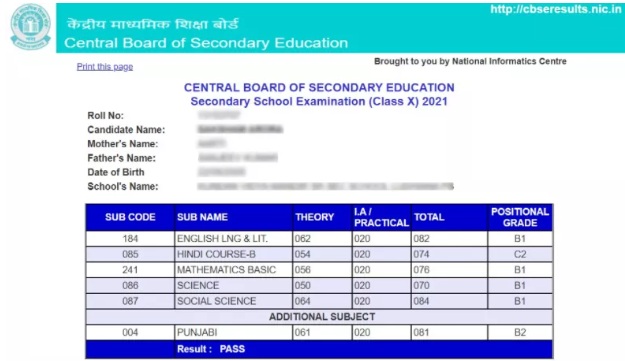## How to Calculate Percentage to CGPA?

If any student gets marks in numeric form and wants to convert Percentage to CGPA, then, he can check the table that is given below to note the Grades he got in each subject.## How to convert CGPA into a Percentage out of 4?

Cumulative Grade Point Average is the name of the calculation used to calculate CGPA. It's a statistic used in schools and colleges to evaluate students' overall academic achievement. This rating scale was developed by the Central Board of Secondary Education.

The conversion of CGPA to percentage out of 4 provides standardized measures of various degrees of accomplishment in a course. A grade can be given in the form of letters, a range, a percentage, or a number that is subtracted from a potential total to find out the cgpa to percentage.

The Steps to Convert CGPA to Percentage out of 4

Considering that the mentioned students' CGPA is 4,

To get the estimated percentage, multiply the obtained CGPA by 9.5.

So, 4x9.5= 38%

Hence, a student's percentage achievement is 38%

## CGPA to Percentage for Engineering

Many Universities in India follow a 4 Scale grading system to distribute marks among the students of  Engineering. Whereas many universities follow their own grading system. In such cases, the University formula is used to know the percentage of students.

(i) Formula which is being used by AICTE and IIT Madras presently:
Percentage = 10 × CGPA − 7.5,
equivalently, Percentage = 52.5 + 10 (CGPA − 6).
(ii) Formula which is being used by some NITs1
and CUSAT:
Percentage = 10 × CGPA − 5,
equivalently, Percentage = 55 + 10(CGPA − 6).

## Percentage to GPA converter

When it comes to comprehending the distinction between GPA and CGPA, students frequently struggle, especially when applying for UG-Undergraduate or PG-Postgraduate programmes (in certain situations). The percentage to GPA converter method is widely used to determine grades in India, which is the main cause of this. Even though how to convert CGPA to percentage is still fairly confusing, the CGPA technique of assessing a student's academic achievement has grown in favor of India's present educational system.

To convert Percentage(%) to GPA, there are two basic scales that are frequently used, a 10.0 scale or a 4.0 scale. The US uses a 4.0 scale, while India uses a GPA scale of 10.0. Students must divide their percentage(%) by 100, multiply the result by 4, and then divide the result by 4 again to obtain their GPA on a 4.0 scale. The formula to convert a percentage to a GPA on a scale of 10.0 and 4.0 are as follows:

Percentage(%) to GPA on 10.0 Scale - Percentage-%/9.5

Percentage(%) to GPA on 4.0 Scale - (Percentage-%/100)x4

### how to convert gpa into marks

Nowdays GPA have been included in various jobs and posting such as in SSC exam but there are still some exams where marks would be the only consideration than, there are many free tools available online which helps in convert GPA into Marks. For example, if a student scores GPA 10 then the average marks student is scoring is 550 and if student still didnt understand how to convert CGPA to Percentage?then they can refer to any free tool for that.

A+97-100

4.0

A93-96

4.0

A-90-92

3.7

B+87-89

3.3

B83-86

3.0

B-80-82

2.7

C+77-79

2.3

C73-76

2.0

C-70-72

1.7

D+67-69

1.3

D65-66

1.0

E/FUnder 650.0

## How to Calculate CGPA into Percentage Delhi University:

DU Cut Off List has been released on 19 October 2023 for the undergraduate courses and 1st Cut off list last date is 21 October 2023. More information is available on the official website of the university. On 11 January 2018, The University of Delhi announced, officially the conversion formula for Cumulative Grade Point Average. The CGPA to percentage formula is the same for all courses. Until now, the University did not specify any particular formula for conversion, which confused students applying for opportunities in India and across the world.

In Delhi Universities, the formula for Delhi University CGPA to percentage under CBCS was made on the Website in 2018. Since that, Delhi University has not specified any particular formula for students applying around the world.

Delhi University asks Percentage in the DU admission application form. It is a difficult task for students to calculate their percentage from CGPA. There is a specific formula available to calculate the percentage from the CGPA Grade. Students need to multiply the grade point they got with 9.5. The percentage for each grade point is mentioned on the page. Check it to know the correct percentage or CGPA.

As per the notice, the formula for CGPA to Percentage for DU (Delhi University) is the Final percentage of marks = 9.5 x CGPA of all six semesters.

### LPU CGPA to Percentage

LPU full form is Lovely Professional University a private university that uses GPA to grant admission. GCPA is different from GPA.  GPA  is used internationally all over the world. After completing any UG degree your marks will be evaluated in GPA form. CGPA 10-pointers are commonly used in LPU and many other universities in India.

KTU CGPA into Percentage Calculator

APJ Abdul Kalam Technological University (KTU) uses CGPA Pattern to distribute marks. The University shows Sum of (Ci*GPi)/Sum of (Ci).

How to Calculate CGPA into Percentage in Vidyasagar University?

(a) In the case of an annual system, the percentage (%) of marks will be calculated on the basis of
UG (Hons) marks were obtained (Part-I, Part-II & Part-III taken together).
(b) In the case of the semester (CBCS) system, UG (Hons) equivalent percentage of marks converted from CGPA based on all semesters ( Sem-I to Sem-VI),as per the rule fixed by their
respective home institution.
(i) For the Vidyasagar University semester system (CBCS):
Let, CGPA = 7.1, The equivalent percentage of marks for V.U. students (as per conversion
rule of V.U) = 7.1 ×10 = 71
(ii) For other institutions with a semester system (CBCS):
Similarly, for the students of other universities/Institutions and Autonomous Colleges
affiliated to V.U, percentage of marks will be calculated as per the percentage conversion rule fixed by their home University/ Institution and Colleges

### CGPA to percentage Mumbai University

Mumbai University changed the CGPA to a Percentage conversion system in 2018.

According to requests received from applicants and other institutions on the conversion of the cumulative grade point average to percentage, the departments of the Mumbai University have made this known. The committee was established by the University with attention for the prerequisites in order to create the formula.

The cumulative Grade Point Average (CGPA) is computed using a weighted average approach, which makes it impossible to properly change the CGPA to the actual percentage of marks.  The conversion formula is an approximate representation of CGPA to Percentage(%).

• For 7 Point Grading System (CGBS),The percentage shall be calculated on the percentage of actual marks obtained by the students.
• For the 10 Point Grading System (CBCS),the formula for calculating the percentage is given by-

Percentage (%) = 7.1 x CGPA + 11

### How to Calculate CGPA into Percentage Visvesaraya Technological University (VTU):

VTU has a different method to Calculate CGPA to Percentage and uses a 7 grading point system. The CGPA gets Subtracted by 0.75 and multiplied by 10.

-> The formula to convert CGPA to percentage is: Percentage = [CGPA-0.75] * 10

### How to Calculate CGPA into Percentage Dayalbagh Educational Institute (DEI):

DEI calculates grades on the 11 Point Scale. Candidates are awarded SGPA every semester by average Grade Points (GP) in the Credit (C) ratio of each Course. The Procedure for Calculating SGPA:

SGPA = GP1 x C1 + GP2 x C2 + ………. + GPn x Cn / C1 + C2 + …….. + Cn

The Overall performance of all semester programs is given as CGPA, a Similar way for the subject's credit during the entire program. Multiplication of CGPA by 10 equivalent marks of all semester.

The minimum CGPA for different Programme is given in the following table and is common for both PG and UG Programmes:

 S.No Division CGPA 1. First with Distinction 8.5 2. First 6 3. Second 4.5

Also check out:Dayalbagh Educational Institue admission 2023

### How to Calculate CGPA into Percentage for Gujarat Technological University (GTU Percentage Calculator)

In GTU students get grades like SPI(Semester Performance Index),CPI (Cumulative Performance Index),and CGPA (Cumulative Grade Point Average). Students can have a semester-based performance by using SPI grades, CPI grades are used to know the overall performance of the year. In the last the CGPA is used to show overall performance in the specific course. CGPA full form in GTU is Cumulative Grade Point Average. This system is new in India.

CGPA To Percentage in Gujarat Technological University (GTU),the Formula of it is:

Percentage = (CGPA-0.5) *10

### How to Calculate CGPA into Percentage in Anna University

Students could indeed find more information in the article that follows. This article will demonstrate how to use Anna University's percentage calculator to convert CGPA to Percentage(%). This CGPA to % conversion would be beneficial to students studying engineering, business administration, and commerce as well as MCA, MBA, and M.Sc students.

Results are proclaimed by Anna University Chennai in accordance with the overall performance of the students. A student will be given the skill based on their estimated CGPA.

CGPA: Stands for Cumulative Grade Point Average

SGPA: Stands for Semester Grade Point Average (Performance of a student in a Sem.

How To Calculate CGPA to Percentage(%)

The algorithm for conversion CGPA to Percentage(%) is mentioned below for your reference. Your CGPA may be manually calculated and converted to a percentage.

Formula: Percentage of Marks = CGPA X 10

Simply multiply your CGPA by 10 to determine the percentage(%).

-> Used standard method multiplying your CGPA * 10

### How to Calculate CGPA into Percentage Chandigarh University CGPA To Percentage Rule

Chandigarh university uses a CGPA system to evaluate the marks of its students. Each semester grades are awarded to students on the basis of performance. The highest marks are considered as a reference to allot grade points.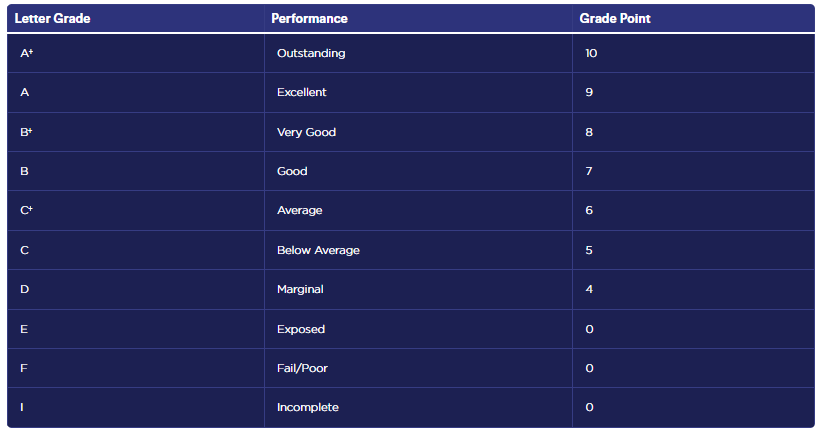### how to calculate cgpa into percentage in APJ Abdul Kalam Technological University (AKTU):

AKTU has a unique procedure for calculating CGPA to Percentage, which Uses a 10 point grading system. You have to remember a few things before CGPA to Percentage. The Formula to calculate CGPA is CGPA= Sum of (Ci*GPi)/ Sum of (Ci), Ci= credit given by to a particular course, GPi= Grade point on courses, and you need to include failed courses also. For Percentage calculation, the Formula is: Percentage = 10 * CGPA – 3.75

### How to Convert CGPA into Percentage in Amity university

Many students get confused about CGPA. As they don't know how to convert cgpa into a percentage for amity university admission.

has a very simple method to calculate CGPA to Percentage for semester exams and Campus Recruitment:

 CGPA  * 10 = Percentage (%)

For Example: If somebody is having 5.7 CGPA till the current semester then his/her percentage would be

 5.7  X  10  =   57%

### CGPA to percentage SPPU

According to the decision made by the SPPU university authorities, the following information is provided in response to several inquiries regarding the conversion of CGPA to percentage for the fulfillment of requirements needed to apply for various vacancies in the Central and State Governments sector, the Defense establishment, etc.

The conversion of CGPA into percentage of marks be calculated as pr following formula-

Percentage(%) of marks = CGPA x 8.80

where CGPA = Sum of Grade points + Credits for each Course

Total Credits

This conversion should be done at the college level and given to the students with the principal's certification in the format specified in the attachment.

CGPA to percentage conversion for D Grade

Percentage(%) = CGPA x 6.6 + 13.6

CGPA to percentage conversion for C Grade

Percentage(%) = CGPA x 10 - 2.50

CGPA to percentage conversion for B Grade

Percentage(%) = CGPA x 10 - 2.50

CGPA to percentage conversion for B+ Grade

Percentage(%) = CGPA x 5 - 26.25

CGPA to percentage conversion for A Grade

Percentage(%) = CGPA x 10 - 7.5

CGPA to percentage conversion for A+ Grade

Percentage(%) = CGPA x 12 - 25\

CGPA to percentage conversion for O Grade

Percentage(%) = CGPA x 20 - 100

### how to calculate cgpa into percentage Guru Gobind Singh Indraprastha University (IPU):

has a different Grading system; performance in every semester will be mentioned as Semester Grade Point Average (SGPA)& rounded to 2 decimal digits. All the semesters' cumulative performance will be known as Cumulative Grade Point Average (CGPA).

The formula for calculation of SGPA and CGPA is given below:

 SGPA= C i G i / Ci
 CGPA= C ni G ni / C ni

Ci – number of credits for the ith course.
Gi– grade point obtained in the ith course.
Cni – number of credits of the ith course of the nth semester.
Mni – marks of the ith course of the nth semester.
Gni – grade points of the ith course of the nth semester.

 CGPA of 4.00 – 4.99 shall be placed in the Third Division. CGPA of 5.00 – 6.49 shall be placed in the Second Division. CGPA of 6.50 or above shall be placed in the First Division.

calculation of CGPA to Percentage: The CGPA x 10 shall be deemed equivalent to a percentage of marks obtained by the student for equivalence to the percentage of marks.

### How to Calculate CGPA into percentage in JNTUH

The formula is the same as CBSE to calculate the percentage to CGPA or vice versa.  JNTUH follows the same formula to generate grade points from percentages. The formula is given below to convert the grade point to CGPA.  Students who have to fill their CGPA to Percentage easily by using this formula.

% of Marks = (final CGPA – 0.5) x 10

### Jamia Millia Islamia:

Jamia Millia Islamia has a unique way to calculate CGPA to Percentage

The Semester Grade Point Average (SGPA) will be computed using the following Formula:

-> SGPA = Σ [ Gn .Crn] / Σ Crn

where Gn is the grade point awarded for the nth course and Crn is the number of credits assigned to the nth course. The summation in the above extends to the total number of courses in the semester. Computation of SGPA would be done up to the second decimal place

The Cumulative Grade Point Average (CGPA) of a student will be computed using the Formula:

-> CGPA=Σ {[ (SGPA)1.S1]+ Σ [ (SGPA)2.S2]}/(S1+S2)

where (SGPA)1 and (SGPA)2 are the SGPA of the 1st and 2nd semesters, and S1 and S2 are the total credits for the 1st and 2nd semesters.

### How to Convert CGPA into Percentage in Delhi Technological University:

In a semester, each curriculum is given a certain number of academic credits. These credits are indeed very important in determining your CGPA, or cumulative grade point average. Students will input the grade points or these credit points into the Delhi Technological University Percentage(%) calculator to determine your final percentage(%) from CGPA.

According to the Delhi Technology University's 2022 grading system requirements, this is the percentage choice for the appropriate CGPA points. Students can use this to compare their percentage(%) determined using the DTU Percentage(%) calculator.

Converting CGPA to Percentage(%)

The CGPA of DTU graduates can theoretically be multiplied by a factor of 10 to convert it to Percentage(%).

Percentage(%) = CGPA x 10

Take the CGPA score from the first scenario as an example. Now, its equal in percentage is,

Percentage(%) = 6.745 X 10 = 67.45%

-> Marks in Percentage = CGPA*10

### Vardhaman Open University CGPA System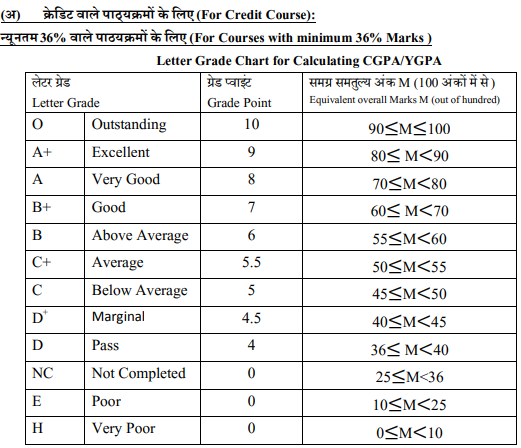In VMOU SGPA is used to calculate the Semester Grade points the formula is mentioned below whereas, the yearly-based Grade points are denoted as YGPA. The year's Grade point is compiled as CGPA In VMOU.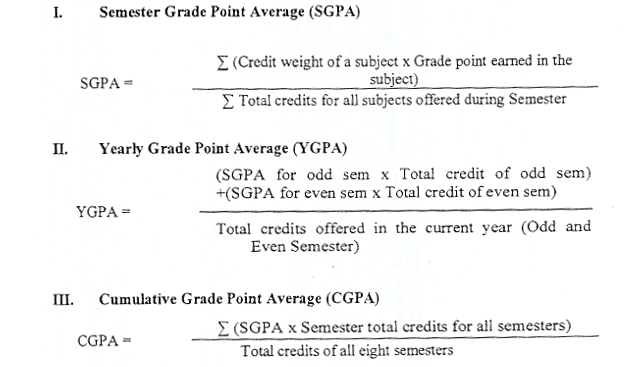### How to Calculate CGPA into Percentage for AKTU (Dr. A.P.J. Abdul Kalam Technical University)

The conversion formula for the conversion of CGPA into Percentage at AKTU is (CGPA-0.75) x 10 = Percentage of marks scored.

CGPA to Percentage: (7.66-0.75) x 10 =69.1%

### SRM University CGPA TO Percentage

SRM University has different rules to convert CGPA to Percentage. SRM University for providing the CGPA and SGPA point grading scales. SRM Authorities convert the CGPA to a percentage while compiling the grade cards following evaluation. Students' exams results are the most important consideration for every admissions committee when determining your eligibility to apply for any discipline.

Let's have a quick glance at the calculators used in each discipline and how SRM University converts CGPA to Percentage.The cumulative grade point average abbreviated as CGPA is calculated by dividing the grade points achieved by the total number of credits taken. The marks will be assigned based on the average of the grades you receive from the SRM University evaluators.

Those candidates who are looking for how to convert CGPA to Percentage must multiplied by 9.5 to be expressed as a percentage(%).

For example, use the following simple and streamlined procedures to integrate your CGPA to a percentage(%):

• Divide the proportion of subjects from the total grade points.
• To convert the CGPA to a percentage(%),multiply it by 9.5.

What Do The Abbreviations Found In Results Mean?

 ABST: Absent EIOP: Eligible for Improvement of Performance N.E: Not Eligible N.R: Not Registered NIOP: Not Eligible for Improvement of Performance QUAL: Qualified for Admission to Higher Classes R.W: Result Withheld SJD: Subjudice TRNS: Transfer Case UFM: Unfair Means XXXX: Appeared for Upgradation of Performance/ Additional Subject

### How to Calculate CGPA into Percentage: OSOU CGPA Calculator

Odisha State Open University (OSOU) students search for CGPA Calculator to know their percentage. because many times it is asked in the form of a percentage. At that, they need a CGPA Calculator. It is very easy to calculate percentages if any student knows his grade points. The formula is given below.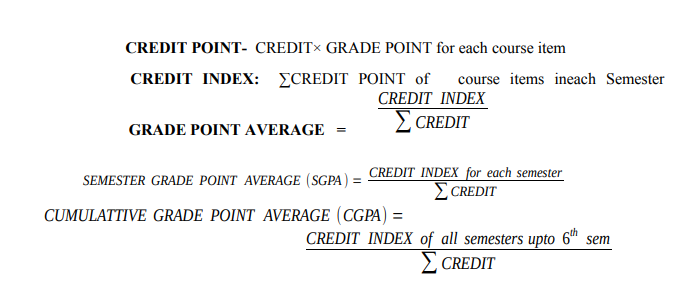## How to Calculate CGPA into Percentage for Engineering Courses

The calculation is different for Engineering courses in India. Each university awards the scores in a different pattern. Many universities use 4 point grading system on the other hand many use 10 Point grading system to distribute marks among students. Many students get confused as they do not know how to convert CGPA to percentage or vice versa.

how to convert cgpa into a percentage out of 4?

Many candidates are confused about how to calculate the cgpa into percentages here is a simple way to solve the problem engineering students can check the grade they get and in the next column to it, the Percent scale is mentioned.

### Advantages Of The CGPA System

1. The very first advantage is, the grading system has lowered the pressure on the students to target for higher marks because, in the grading system, actual marks are not written on the card. Hence, students are not bothered about getting good marks, and they need to focus on grades only.
2. The second advantage that the grading system has is that it helps teachers classify the students as bright, average, and below. So, they can focus on average and below students to make them bright students.
3. This new education system helps Indian parents to know about their children’s other capabilities. Like some students are not good in studies but they are outstanding in art and crafts.

4. CGPA system reduces the pressure of scores and competition among students and helps them to develop a helping nature that will also help them in the future.

5. The grading system is all dependent upon advanced patterns, as in this system, grades are not only calculated based on marks. It also consists of achievement, extracurricular activities, academic performance, attendance, etc.
6. CGPA was introduced in 2011 in the Indian education system to reduce stress levels due to high competition among students. The main purpose of the CGPA system is to provide quality education and help new learners to think creatively.

7. It helps to develop other skills like communication, presentation, and critical thinking.

### Percentage to CGPA: Conversion Table

 Percentage CGPA (Equivalent) Percentage CGPA (Equivalent) 39.9 4.2 68.4 7.2 40.85 4.3 69.35 7.3 41.8 4.4 70.3 7.4 42.75 4.5 71.25 7.5 43.7 4.6 72.2 7.6 44.65 4.7 73.15 7.7 45.6 4.8 74.1 7.8 46.55 4.9 75.05 7.9 47.5 5 76 8 48.45 5.1 76.95 8.1 49.4 5.2 77.9 8.2 50.35 5.3 78.85 8.3 51.3 5.4 79.8 8.4 52.25 5.5 80.75 8.5 53.2 5.6 81.7 8.6 54.15 5.7 82.65 8.7 55.1 5.8 83.6 8.8 56.05 5.9 84.55 8.9 57 6 85.5 9 57.95 6.1 86.45 9.1 58.9 6.2 87.4 9.2 59.85 6.3 88.35 9.3 60.8 6.4 89.3 9.4 61.75 6.5 90.25 9.5 62.7 6.6 91.2 9.6 63.65 6.7 92.15 9.7 64.6 6.8 93.1 9.8 65.55 6.9 94.05 9.9 66.5 7 95 10

Question 1. What is the full form of CGPA?

What exactly is CGPA?Answer: CGPA is a grading system that has been used in the educational sector. Cumulative Grade Points are the average of grade points obtained in all subjects.

Question 2. How do I calculate CGPA to Percentage?

Answer: If you want to calculate the percentage from your CGPA, then you simply multiply your CGPA by 9.5.

For example: if you’re CGPA is 9.8

Just do 9.8×9.5 =93.10% (It is your Marks in percentage)

Question 3. How can I convert CGPA to Percentage?

Answer: If you want to convert CGPA from your percentage, then you simply divide it by 9.5

For example: if your percentage is 93.10%

Just do 93.10/9.5= 9.8 (it’s your CGPA)

Question 4. How to Convert 3.55 CGPA to Percentage?

Answer: If you want to Convert CGPA from Percentage Simply 3.55*9.5 i.e., 33.73%

Question 5. How to convert CGPA into a percentage out of 4

Answer: Just do 4 x 9.5= 38%

 4 CGPA 38%

#### Question 6. How to Calculate CGPA?

Answer: Calculate CGPA by adding the marks of the main subject and dividing the sum obtained by the total number of subjects then you get CGPA.

Question 5. 8.64 CGPA to a percentage?